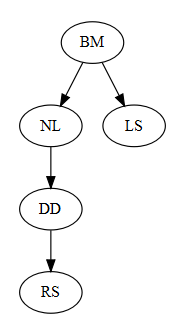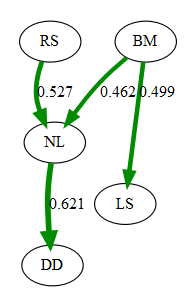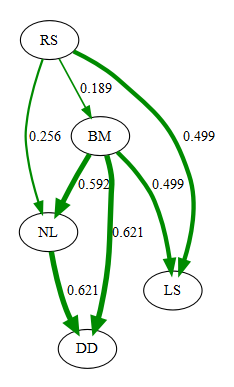# Introduction to phylogenetic path analysis with phylopath

#### 2016-10-04

This vignette gives a quick overview of the package by recreating the phylogenetic path analysis described in:

Gonzalez-Voyer A & von Hardenberg A. 2014. An Introduction to Phylogenetic Path Analysis. Chapter 8. In: Garamszegi LZ (ed.), Modern Phylogenetic Comparative Methods and Their Application in Evolutionary Biology. pp. 201-229. Springer-Verlag Berlin Heidelberg.

You can find this book chapter online. For an introduction to the methodology, as well as the data, see the wonderful book chapter.

Specifically, we recreate the Rhinogrades example here. The data used has been included in this package.

Following figure 8.7, we first create all 9 causal models using the `DAG` function. This function uses regression equations (or `formula`s) to express the hypothesized relationships in the models. The easiest way to create this is by taking each node (a variable), putting it on the left-hand side of a tilde (`~`), and putting its causal parents on the right-hand side.

Note: any unconnected nodes can be added using `var ~ var`, but we won’t be needing that for this example.

``````library(phylopath)

models <- list(
one   = DAG(LS ~ BM, NL ~ BM, DD ~ NL, RS ~ DD),
two   = DAG(LS ~ BM, NL ~ BM, DD ~ NL, RS ~ LS + DD),
three = DAG(LS ~ BM, NL ~ BM, DD ~ NL, RS ~ NL),
four  = DAG(LS ~ BM, NL ~ BM, DD ~ NL, RS ~ BM + NL),
five  = DAG(LS ~ BM, NL ~ BM, DD ~ NL, RS ~ BM + NL + DD),
six   = DAG(LS ~ BM, NL ~ BM + RS, DD ~ NL, RS ~ BM),
seven = DAG(LS ~ BM, NL ~ BM + RS, DD ~ NL, RS ~ LS + BM),
eight = DAG(LS ~ BM, NL ~ BM + RS, DD ~ NL),
nine  = DAG(LS ~ BM, NL ~ BM + RS, DD ~ NL, RS ~ LS)
)``````

The `DAG` function simply produces a matrix that summarizes the connections between the variables.

``models\$one``
``````##    BM NL DD RS LS
## BM  0  1  0  0  1
## NL  0  0  1  0  0
## DD  0  0  0  1  0
## RS  0  0  0  0  0
## LS  0  0  0  0  0
## attr(,"class")
##  "matrix" "DAG"``````

Note that the models are of class `matrix` as well as of class `DAG`. This means we can have special DAG `methods`.

For example, it is good to check if the DAG looks like you were expecting. Simply `plot` one of the models to inspect it visually.

``plot(models\$one)``Now that we have the models, we can perform the path analysis using the `phylo_path` function. For this we will need a data set, included in this package as `rhino`, as well as a phylogenetic tree, `rhino_tree`.

Importantly, when using PGLS, we need to be consistent in which variables are used as independent and dependent variables in the analysis. If one has a specific idea about which variables are to be conscidered as up- and down-stream, then you can use the `order` argument to give the ordering (from up to down). In this case, we supply the ordering to mimic the choices made by the chapter authors. Alternatively, you can choose to not supply an order, and the function will try to make a sensible order by itself. If the combination of all causal models is itself a DAG, the ordering of that model will be used, otherwise the ordering will be constructed by consensus (i.e. the most common ordering is chosen).

By default, `phylo_path` uses Pagel’s “lambda” correlation structure (`ape::corPagel`), but if you want, for example, to use a simple Brownian motion model, you can supply `ape::corBrownian` instead.

``````result <- phylo_path(models, data = rhino, tree = rhino_tree,
order = c('BM', 'NL', 'DD', 'LS', 'RS'))``````

The result we end up with is a `phylo_path` object. Simply printing it gives us a quick summary of what is in the object.

``result``
``````##
## A phylogenetic path analysis
##
##   Evaluated for these models: one two three four five six seven eight nine
##
##   Containing 36 phylogenetic regressions.``````

To get an overview of the analysis, we can ask for its `summary`:

``summary(result)``
``````##   model k  q      C     p   CICc delta_CICc     l     w
## 1 eight 6  9  8.200 0.769 28.200      0.000 1.000 0.324
## 2   six 5 10  6.653 0.758 29.125      0.924 0.630 0.204
## 3  four 5 10  6.761 0.748 29.233      1.032 0.597 0.193
## 4  nine 5 10  7.903 0.638 30.375      2.174 0.337 0.109
## 5  five 4 11  5.447 0.709 30.447      2.247 0.325 0.105
## 6 seven 4 11  6.457 0.596 31.457      3.257 0.196 0.064
## 7 three 6  9 29.490 0.003 49.490     21.290 0.000 0.000
## 8   one 6  9 64.371 0.000 84.371     56.171 0.000 0.000
## 9   two 5 10 63.386 0.000 85.858     57.657 0.000 0.000``````

The ranking of the models obtained here is identical as the worked example in the book chapter. The estimates differ slightly however, since we are using `nlme::gls` whereas the chapter was using `caper::pgls`.

To view the best ranked model, we can use `best`. This returns a DAG with standardized regression coefficients, as well matrices of standard errors and confidence intervals. These can be obtained for any particular DAG using `est_DAG`.

``(best_model <- best(result))``
``````## \$coef
##    RS BM        NL        DD        LS
## RS  0  0 0.5272990 0.0000000 0.0000000
## BM  0  0 0.4623828 0.0000000 0.4988607
## NL  0  0 0.0000000 0.6206986 0.0000000
## DD  0  0 0.0000000 0.0000000 0.0000000
## LS  0  0 0.0000000 0.0000000 0.0000000
##
## \$se
##    RS BM         NL         DD         LS
## RS  0  0 0.05721587 0.00000000 0.00000000
## BM  0  0 0.06510549 0.00000000 0.08954569
## NL  0  0 0.00000000 0.08116661 0.00000000
## DD  0  0 0.00000000 0.00000000 0.00000000
## LS  0  0 0.00000000 0.00000000 0.00000000
##
## \$lower
##    RS BM        NL        DD        LS
## RS  0  0 0.4137414 0.0000000 0.0000000
## BM  0  0 0.3331664 0.0000000 0.3211602
## NL  0  0 0.0000000 0.4596261 0.0000000
## DD  0  0 0.0000000 0.0000000 0.0000000
## LS  0  0 0.0000000 0.0000000 0.0000000
##
## \$upper
##    RS BM        NL        DD        LS
## RS  0  0 0.6408567 0.0000000 0.0000000
## BM  0  0 0.5915991 0.0000000 0.6765612
## NL  0  0 0.0000000 0.7817711 0.0000000
## DD  0  0 0.0000000 0.0000000 0.0000000
## LS  0  0 0.0000000 0.0000000 0.0000000
##
## attr(,"class")
##  "fitted_DAG"``````

This object can also be plotted, now the numbers and width of the arrow represent path coefficients. In this case, all paths are green since all relationships are positive.

``plot(best_model)``From the summary we could see that in reality, there are several models that are quite good. Instead of using the best model, we can use the average of the best models, weigted by their relative evidence. By simply calling `average`, we can obtain the coefficients and standard errors of the averaged model where the CICc `cut_off` is 2 by default. If a model does not include a path, we assume that coefficient to be 0.

``average_model <- average(result)``
``plot(average_model)``Note that, by default, the path averaging is only done for the models that actually contain that path. This facilitates the detection of weak effects, but also biases coefficients away from zero. Alternatively, we can assume the coefficients (and their variance) for absent paths to be zero by setting `method = "full"`.

``average_model_full <- average(result, method = "full")``
``plot(average_model_full)``